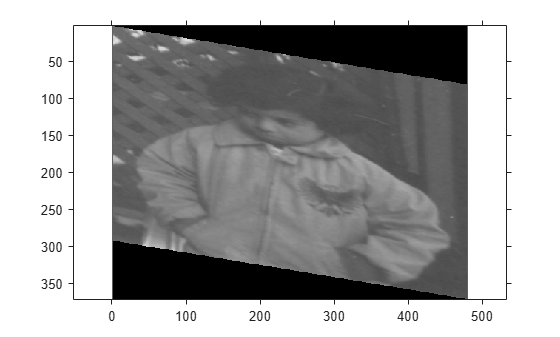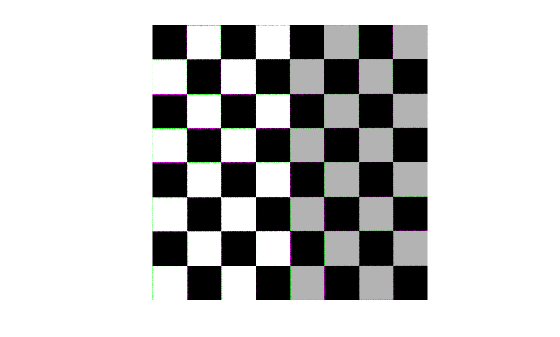# affine2d

2-D affine geometric transformation

## Description

An `affine2d` object stores information about a 2-D affine geometric transformation and enables forward and inverse transformations.

## Creation

You can create an `affine2d` object in these ways:

• `imregtform` — Estimate a geometric transformation that maps a moving image to a fixed image using similarity optimization.

• `imregcorr` — Estimate a geometric transformation that maps a moving image to a fixed image using phase correlation.

• `fitgeotrans` — Estimate a geometric transformation that maps pairs of control points between two images.

• `randomAffine2d` — Create a randomized 2-D affine transformation.

• The `affine2d` function described here.

### Syntax

``tform = affine2d``
``tform = affine2d(T)``

### Description

````tform = affine2d` creates an `affine2d` object with default property settings that correspond to the identity transformation.```

example

````tform = affine2d(T)` sets the property `T` as the specified valid affine transformation matrix.```

## Properties

expand all

Forward 2-D affine transformation, specified as a nonsingular 3-by-3 numeric matrix.

The matrix `T` uses the convention:

`[x y 1] = [u v 1] * T`

where `T` has the form:

``` [a b 0; c d 0; e f 1]; ```

The default of `T` is the identity transformation.

Data Types: `double` | `single`

Dimensionality of the geometric transformation for both input and output points, specified as the value `2`.

## Object Functions

 `invert` Invert geometric transformation `isRigid` Determine if transformation is rigid transformation `isSimilarity` Determine if transformation is similarity transformation `isTranslation` Determine if transformation is pure translation `outputLimits` Find output spatial limits given input spatial limits `transformPointsForward` Apply forward geometric transformation `transformPointsInverse` Apply inverse geometric transformation

## Examples

collapse all

Create an `affine2d` object that defines a 30 degree rotation in the counterclockwise direction around the origin.

```theta = 30; tform = affine2d([ ... cosd(theta) sind(theta) 0;... -sind(theta) cosd(theta) 0; ... 0 0 1])```
```tform = affine2d with properties: T: [3x3 double] Dimensionality: 2 ```

Apply the forward geometric transformation to a point (10,0).

`[x,y] = transformPointsForward(tform,10,0)`
```x = 8.6603 ```
```y = 5 ```

Validate the transformation by plotting the original point (in blue) and the transformed point (in red).

```plot(10,0,'bo',x,y,'ro') axis([0 12 0 12]) axis square```Read an image into the workspace.

`A = imread('pout.tif');`

Create an `affine2d` object that defines an affine geometric transformation. This example combines vertical shear and horizontal stretch.

`tform = affine2d([2 0.33 0; 0 1 0; 0 0 1])`
```tform = affine2d with properties: T: [3x3 double] Dimensionality: 2 ```

Apply the geometric transformation to the image using `imwarp`.

`B = imwarp(A,tform);`

Display the resulting image.

```figure imshow(B); axis on equal;``````I = imread('kobi.png'); imshow(I)```Create an `affine2d` transformation object that rotates images. The `randomAffine2d` function picks a rotation angle randomly from a continuous uniform distribution within the interval [35, 55] degrees.

`tform1 = randomAffine2d('Rotation',[35 55]);`

Rotate the image and display the result.

```J = imwarp(I,tform1); imshow(J)```The transformation object, `tform1`, rotates all images by the same amount. To rotate an image by a different randomly selected amount, create a new `affine2d` transformation object.

```tform2 = randomAffine2d('Rotation',[-10 10]); J2 = imwarp(I,tform2); imshow(J2)```This example shows how to create a geometric transformation that can be used to align two images.

Create a checkerboard image and rotate it to create a misaligned image.

```I = checkerboard(40); J = imrotate(I,30); imshowpair(I,J,'montage')```Define some matching control points on the fixed image (the checkerboard) and moving image (the rotated checkerboard). You can define points interactively using the Control Point Selection tool.

```fixedPoints = [41 41; 281 161]; movingPoints = [56 175; 324 160];```

Create a geometric transformation that can be used to align the two images, returned as an `affine2d` geometric transformation object.

`tform = fitgeotrans(movingPoints,fixedPoints,'NonreflectiveSimilarity')`
```tform = affine2d with properties: T: [3x3 double] Dimensionality: 2 ```

Use the `tform` estimate to resample the rotated image to register it with the fixed image. The regions of color (green and magenta) in the false color overlay image indicate error in the registration. This error comes from a lack of precise correspondence in the control points.

```Jregistered = imwarp(J,tform,'OutputView',imref2d(size(I))); figure imshowpair(I,Jregistered)```Recover angle and scale of the transformation by checking how a unit vector parallel to the x-axis is rotated and stretched.

```u = [0 1]; v = [0 0]; [x, y] = transformPointsForward(tform, u, v); dx = x(2) - x(1); dy = y(2) - y(1); angle = (180/pi) * atan2(dy, dx) ```
```angle = 29.7686 ```
`scale = 1 / sqrt(dx^2 + dy^2)`
```scale = 1.0003 ```

## Version History

Introduced in R2013a